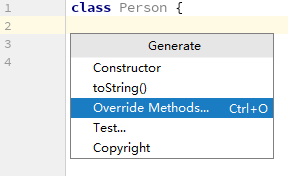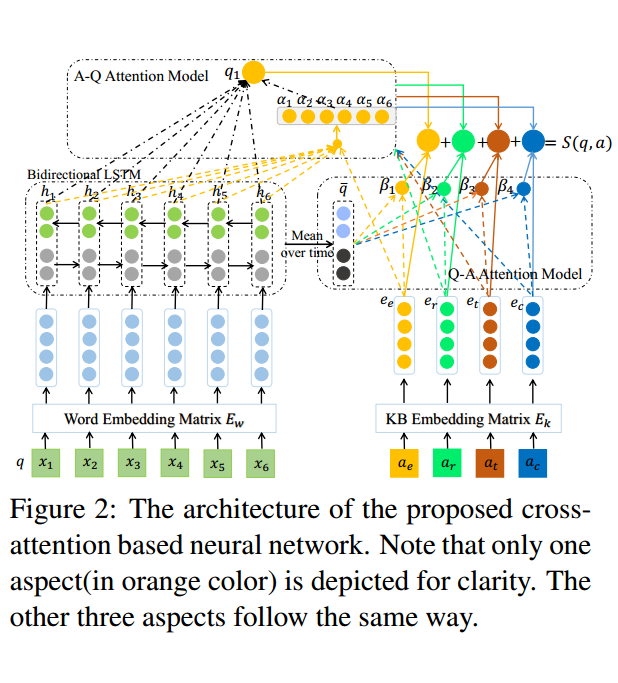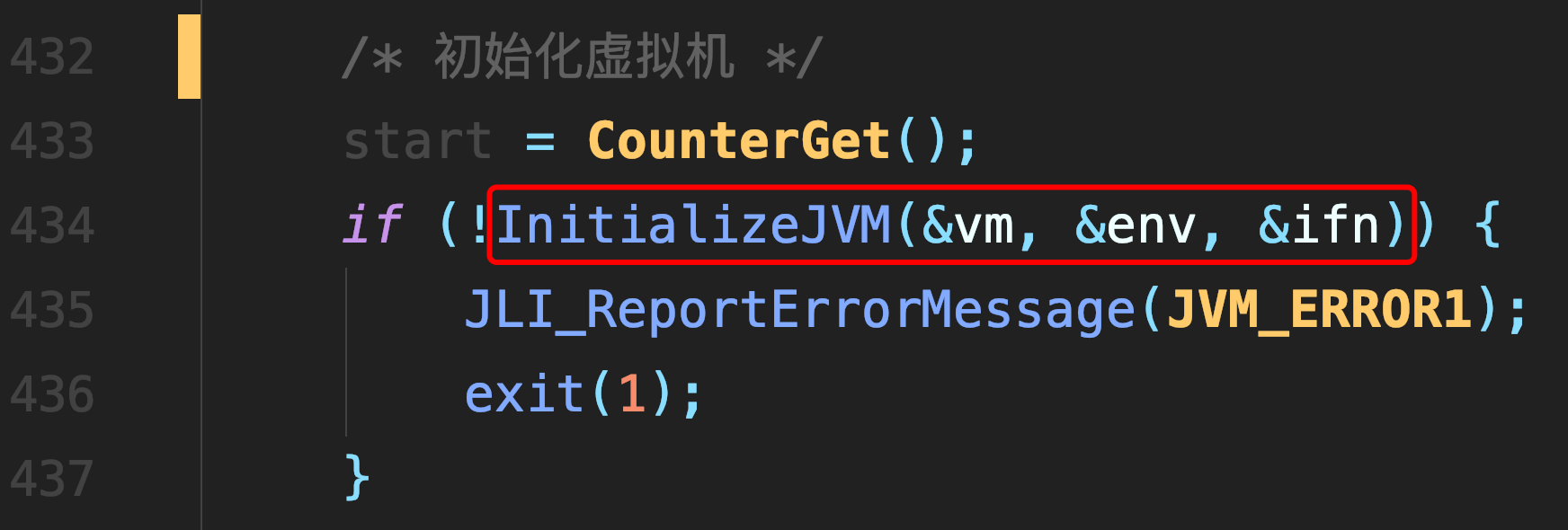# 拉格朗日乘子法

### 拉格朗日乘子法

• 原始问题

假设 $f(x), c_i(x),h_j(x)$是定义在 $R^n$上的连续可微函数。原问题如下(包括约束)
$\mathop{min}_{x\in R_n} f(x)\\ s.t.\ \ \ c_i(x)<=0,\ i= 1,2,..k \\ \ \ \ \ \ h_j(x)=0, \ j=1,2,...l$
引入广义拉格朗日函数 $L(x, \alpha, \beta)=f(x)+\sum\limits_{i=1}^{k}\alpha_ic_i(x)+\sum\limits_{j=1}^{l}\beta_jh_j(x)$

其中 $x\in R^n \ \alpha_i,\beta_j是拉格朗日的乘子$, 特别注意的是 $\alpha_i >= 0$,

考虑 $x$的函数 $\theta_P(x)= \max\limits_{\alpha,\beta:\alpha_i>=0}L(x, \alpha, \beta)$,P代表的是原始问题

其实只要 $x$满足原始问题，则 $\theta_p(x)$就是 $f(x)$，下面会解释

如果 $x$不满足约束条件，即存在 $c_i(x)$>0,则可以使 $\alpha_i \rightarrow +\infty \ ,\theta_P(x) \rightarrow+\infty$,

或者存在 $h_j(x)!=0$,则可以令 $\beta_jh_j(x)\rightarrow+\infty$.(因为只有 $\alpha_i有>=0的要求, \beta_j可以任取正负$)

在满足了约束条件下, $\theta_p(x)==f(x)$,因为 $L(x, \alpha, \beta)$的第三项为零，第二项的最大值也为零

第一项针对变量 $\alpha,\beta$为常数，所以 $\theta_p(x)== \max\limits_{\alpha,\beta:\alpha_i>=0}L(x, \alpha, \beta) ==f(x)$

所以原问题可以等价为 $\min\limits_x\theta_P(x)=\min\limits_x\max\limits_{\alpha,\beta:\alpha_i>=0}L(x,\alpha,\beta)$

$\min\limits_x\max\limits_{\alpha,\beta:\alpha_i>=0}L(x,\alpha,\beta)$称为广义拉格朗日函数的极小极大问题

定义 $p^*=\min\limits_x\theta_P(x)$为原始问题的最优值

• 对偶问题

定义
$\theta_D(x)=\min\limits_xL(x,\alpha,\beta) \\ 再考虑极大化问题即 \max\limits_{\alpha,\beta:\alpha_i>=0}\theta_D(\alpha,\beta)=\max\limits_{\alpha,\beta:\alpha_i>=0}\min\limits_xL(x,\alpha,\beta)$
$\max\limits_{\alpha,\beta:\alpha_i>=0}\min\limits_xL(x,\alpha,\beta)$称为广义拉格朗日函数的极大极小问题

定义 $d^*=\max\limits_{\alpha,\beta:\alpha_i>=0}\theta_D(\alpha,\beta)$,为对偶问题的最优值。

• 原始问题与对偶问题的关系

• 若原始问题和对偶问题都有最优值，则

$d^*=\max\limits_{\alpha,\beta:\alpha_i>=0}\min\limits_xL(x,\alpha,\beta)<=\min\limits_x\max\limits_{\alpha,\beta:\alpha_i>=0}L(x,\alpha,\beta)=p^*$

证明，对于任意的 $\alpha,\beta和x$,有
$\theta_D(\alpha,\beta)=\min\limits_xL(x,\alpha,\beta)<=L(x,\alpha,\beta)<=\max\limits_{\alpha,\beta:\alpha_i>=0}L(x,\alpha,\beta)=\theta_P(x)$
于是得证。

• 推论，设 $x^*,\alpha^*,\beta^*$分别是原始问题和对偶问题的可行解，并且 $d^*==p^*$,则 $x^*,\alpha^*,\beta^*$分别是原始问题

和对偶问题的最优解。在一些情况下，原始问题的和最优问题的最优值相等， $d^*==p^*$,这时可以考虑

用解对偶问题代替解原始问题

• 定理

1. 对于原始问题，假设函数 $f(x)，c_i(x)$是凸函数， $h_j(x)是仿射函数$(即由一阶多项式构成

的函数， $f(x)=Ax + b$, $A$是矩阵， $x，b$是向量)，并且假设不等式约束 $c_i(x)$是严格可行的。

即存在 $x$,对所有 $i$ $c_i(x)<0$,则存在 $x^*,\alpha^*\beta^*$, $x^*$是原始问题的解, $\alpha^*,\beta^*$是对偶问题的解，

并且 $p^*=d^*=L(x^*,\alpha^*,\beta^*)?$

2. 对于原始问题，假设函数 $f(x)，c_i(x)$是凸函数， $h_j(x)是仿射函数$(即由一阶多项式构成

的函数， $f(x)=Ax + b$, $A$是矩阵， $x，b$是向量)，并且假设不等式约束 $c_i(x)$是严格可行的。

即存在 $x$,对所有 $i$ $c_i(x)<0$,则 $x^*,\alpha^*,\beta^*$是原始问题和对偶问题的充分必要条件是，其满足

以下的 $KKT$条件：
$\nabla_xL(x^*,\alpha^*,\beta^*)=0 \\ \alpha_i^*c_i(x^*)=0, i=1,2,...k \\ c_i(x^*)<=0, i=1,2,...k \\ \alpha_i^*>=0, i=1,2,...k (对偶互补条件）\\ h_j(x^*)=0, j=1,2,...l$
开头为什么要函数连续可微，这里就介绍了是为了求函数的偏导

$KKT$对偶互补条件可知，若 $\alpha_i^*>0$,则 $c_i(x^*)=0$.(SVM中用到了这一点)

##### 热门推荐
•浏览(654)
•浏览(563)
•浏览(547)
•浏览(480)
•浏览(424)
•浏览(419)
•浏览(381)
•浏览(366)
•浏览(362)
•浏览(344)